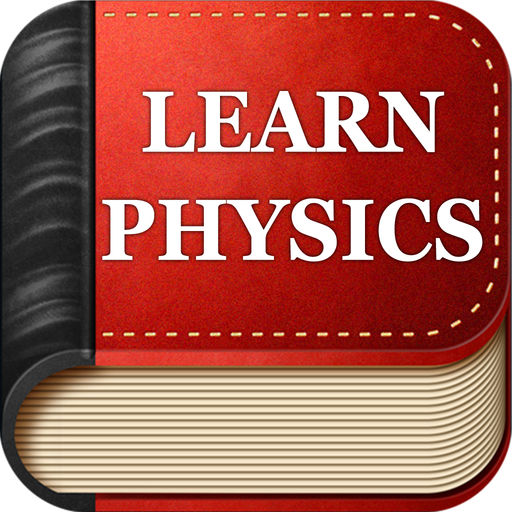iLearnPhysics - Easy way to learn Physics# iLearnPhysics - Easy way to learn Physics

## Paul Cotarlea

5.5

6 Ratings

Release Date

2014-11-19

Size

46.4 MB

### Screenshots for iPhone

iPhone
iLearnPhysics - Easy way to learn Physics Description
This app helps you to understand Physics easily and fast with core concept tutorials, formulas calculator and quizzes.

The app features a comprehensive list of formulas for the topics listed bellow, each with a smart formula calculator that allows you to solve any variable within the formula.

Use the in-app purchase to upgrade to the Pro version for access the additional contents (11 tutorials). In the free version you can access only 4 tutorials.

The list of topics covered by this app:

- MECHANICS -

1. Motion in One Dimension
Section 1. Distance and Displacement
Section 2. Speed and Velocity
Section 3. Position-time graph and Velocity-time graph
Section 4. Acceleration
Section 5. Equations of Motion

2. Forces and Newton's Laws
Section 1. What is a Force
Section 2. Newton's First Law of Motion
Section 3. Newton's Second Law of Motion
Section 4. Newton's Third Law
Section 5. Weight and Normal Forces
Section 6. Friction Force

3. Forces in Two Dimensions
Section 1. Break down forces into components
Section 3. Free body diagram

4. Free Fall and Projectile Motion
Section 1. Free fall
Section 2. Projectile Motion
Section 3. Horizontal Projection
Section 4. Angular Projection

5. Momentum and Collisions
Section 1. Momentum
Section 2. Impulse
Section 3. Impulse-Momentum Theorem
Section 4. Conservation of Momentum
Section 5. Types of Collisions

6. Circular Motion and Rotation
Section 1. Arc Length and Radian Measure
Section 2. Uniform Circular Motion
Section 3. Centripetal and Centrifugal Forces
Section 4. Rotational Motion

7. Mechanics of Solids and Fluids
Section 1. Fluids and Solids: Fundamentals
Section 2. Mechanical Properties of Solids
Section 3. Mechanical Properties of Fluids
Section 4. Pascal's principle (Pascal's law)
Section 5. Archimedes' principle

- ELECTROMAGNETISM -

1. Electrostatics
Section 1. Net Electrical Charge
Section 2. Conductors and Insulators
Section 3. Charged Objects
Section 4. Coulomb's Law

2. Circuit Elements
Section 1. Electric Field
Section 2. Electric Potential Difference
Section 3. Flow of Charge: The Current
Section 4. Passive Circuit Elements
Section 5. Active Circuit Elements

3. Electric Circuits
Section 1. Ohm's Law
Section 2. Kirchhoff's current law (KCL)
Section 3. Kirchhoff's voltage law (KVL)
Section 4. Series Circuits
Section 5. Parallel Circuits
Section 6. Series-Parallel Combination Circuits

4. Magnetism
Section 1. Magnetic Field
Section 2. The Earth’s Magnetic Field
Section 3. Magnetic Force on Moving Charges
Section 4. Magnetic Field from a Wire

5. Waves and Sound
Section 1. The Nature of Waves
Section 2. Wave Properties
Section 3. The Behavior of Waves
Section 4. The Nature of Sound
Section 5. Sound Properties
Section 6. The Doppler Effect

6. Optics
Section 1. The Electromagnetic Spectrum
Section 2. Luminous and Illuminated Bodies
Section 3. Reflection of Light Waves
Section 4. Refraction of Light Waves
Section 5. Concave and Convex Mirrors
Section 6. Convex and Concave Lenses

- THERMODYNAMICS -

1. Energy, Work and Heat
Section 1. System, Surroundings and Boundary
Section 2. Closed, Open and Isolated Systems
Section 3. Intensive and extensive properties
Section 4. States and Processes
Section 5. Energy
Section 6. Work
Section 7. Heat

2. Laws of Thermodynamics
Section 1. Zeroth Law of Thermodynamics
Section 2. First Law of Thermodynamics
Section 3. The Second Law of Thermodynamics
Section 4. The Third Law of Thermodynamics

** DO NOT USE AS CHEAT SHEET ON AN EXAM IF IT IS NOT ALLOWED.

*** REPORT A PROBLEM
If you have any kind of problem please write to our SUPPORT and do not post negative reviews as we cannot respond directly to reviews.
iLearnPhysics - Easy way to learn Physics 1.0.1 Update
2014-11-19 Version History
iOS 8 compatibility
More
Price:
Free
Version:
1.0.1
Size:
46.4 MB
Release Date:
2014-11-19
Developer:
Paul Cotarlea
Language:
English
More
In-App Purchases: